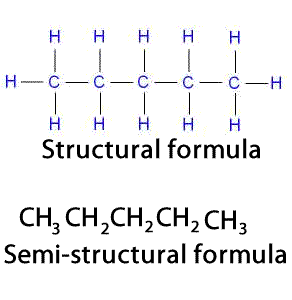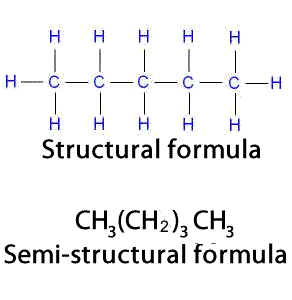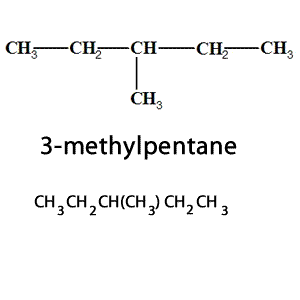Semi-structural formulae In organic chemistry a simple molecular formula is not always enough to identify the compound. Structural and semistructural formulae are needed to identify a compound. Semi -structural formulae are also known as condensed structural formulae. The use of semi-structural formulae is best suited to non-cyclic carbon compounds. Semistructural formulae are always written from left to right one carbon at a time. Lets see how the condensed structural formula of pentane is written. When a compound is represented using its condensed structural formula format, each carbon atom and the hydrogen atoms attached to it are listed as a molecular formula.Still further compression of the formula can be made by placing repeating groups in parentheses and using a subscript to show the number of repeating groups, as shown on the right.Branched groups are shown in brackets following the carbon atom to which they are bonded to. The condensed structural formula on the right shows that in 3-methylpentane, there is a branched, methyl group, attached to the third carbon atom of the pentane chain. What do you think the condensed structural formula of 3-ethylpentane is? What is the condensed structural formula for 2,2,4-Trimethylpentane is? What is the name of the compound given by the condensed structural formula (CH3)2CH(CH2)4CH3 What is the condensed structural formula of 3-ethyl-2-methylheptane? What is the condensed structural formula of 2-aminobutane?